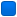###Author Topic: Loop unrolling : crash ?  (Read 3365 times)

#### andre104

• Guest##### Loop unrolling : crash ?
« on: February 16, 2007, 07:09:22 am »
Recently I read an article about loop unrolling (http://en.wikipedia.org/wiki/Loop_unrolling). So I gave a try (but first in Python  :mrgreen: ). The problem is how to change all 100 items in array from 1 t0 0

Without loop unrolling :
Code: [Select]
`import timearr = []for x in range(0,1000000): arr.append(1)start = time.clock()for x in range(0,1000000): arr[x] = 0 finish = time.clock()print finish-start`

With loop unrolling
Code: [Select]
`arr = []for x in range(0,1000000): arr.append(1)start = time.clock()for x in range(0,1000000,5): arr[x] = 0 arr[x+1] = 0 arr[x+2] = 0 arr[x+3] = 0 arr[x+4] = 0 finish = time.clock()print finish-start`

Both worked as already expected. So I port them to C, and crash  :shock:

Without loop unrolling
Code: [Select]
`#include <stdio.h>#include <time.h>#define MAXSIZE 1000000int main(){ clock_t start,finish; long arr[MAXSIZE]; for (long x = 0; x < MAXSIZE; x++) arr[x] = 1; start = clock(); for (long x = 0; x < MAXSIZE; x++) arr[x] = 0; finish = clock(); printf("%f\n",(float)((finish-start)/CLOCKS_PER_SEC)); return 0;}`

With loop unrolling

Code: [Select]
`#include <stdio.h>#include <time.h>#define MAXSIZE 1000000int main(){ clock_t start,finish; long arr[MAXSIZE]; for (long x = 0; x < MAXSIZE; x++) arr[x] = 1; start = clock(); for (long x = 0; x < MAXSIZE; x++) { arr[x] = 0; arr[x+1] = 0; arr[x+2] = 0; arr[x+3] = 0; arr[x+4] = 0; } finish = clock(); printf("%f\n",(float)((finish-start)/CLOCKS_PER_SEC)); return 0;}`

####AlexN

• Global Moderator
• Member
•• Posts: 387##### Re: Loop unrolling : crash ?
« Reply #1 on: February 16, 2007, 08:22:06 am »
Quote from: "andre104"
int main()
{
clock_t start,finish;
long arr[MAXSIZE];

for (long x = 0; x < MAXSIZE; x++) arr
• = 1;

start = clock();

for (long x = 0; x < MAXSIZE; x++) {
arr
• = 0;

arr[x+1] = 0;
arr[x+2] = 0;
arr[x+3] = 0;
arr[x+4] = 0;
}

finish = clock();

printf("%f\n",(float)((finish-start)/CLOCKS_PER_SEC));

return 0;
}
[/code]

You don't unrool the loop, because you increase the loop-counter each time with 1 and write so in each array-cell up to 5 times. When you reach the end of the array you write 0 to the next 4 longs behind the array and this may cause a crash.
best regards
Alex#### andre104

• Guest##### Loop unrolling : crash ?
« Reply #2 on: February 16, 2007, 08:47:41 am »
uuhh ... okay  #-o
so anyway, how should I fix that ?

####AlexN

• Global Moderator
• Member
•• Posts: 387##### Loop unrolling : crash ?
« Reply #3 on: February 16, 2007, 09:02:49 am »
Quote from: "andre104"
uuhh ... okay  #-o
so anyway, how should I fix that ?

The first part is easy. You must  increase the loop-counter by 5.
for (long x = 0; x < MAXSIZE; x+=5)

In this case it would be enough (1000000 / 5 has no rest). But normally you must check if you can make all 5 (in this case) instructions and if not do the rest in a normal loop;
best regards
Alex